# Electrochemistry Class 12 Chemistry Notes

Electrochemistry Class 12 Chemistry notes With formulas, important questions, MCQs, solved numerical problems, and a PDF for NEB Chemistry exam 2080.
Electrochemistry course content
5.0 Origin of Electrodes
5.1 Standard electrode (redox ) potential.
5.2 Standard hydrogen electrodes and calomel electrodes
5.3 Calculate a standard cell potential
5.4 Application of electrochemical series
5.5 Standard cell potential with reference to voltaic cell: Zn-Cu cell, Ag-Cu cell
5.6 Standard cell potentials to explain/deduce the direction of electron flow in a simple cell and predict the feasibility of a reaction
5.7 Cell potential and free energy change
5.8 Commercial Cells, e.g. hydrogen/oxygen, fuel cell, lithium-ion, rechargeable batteries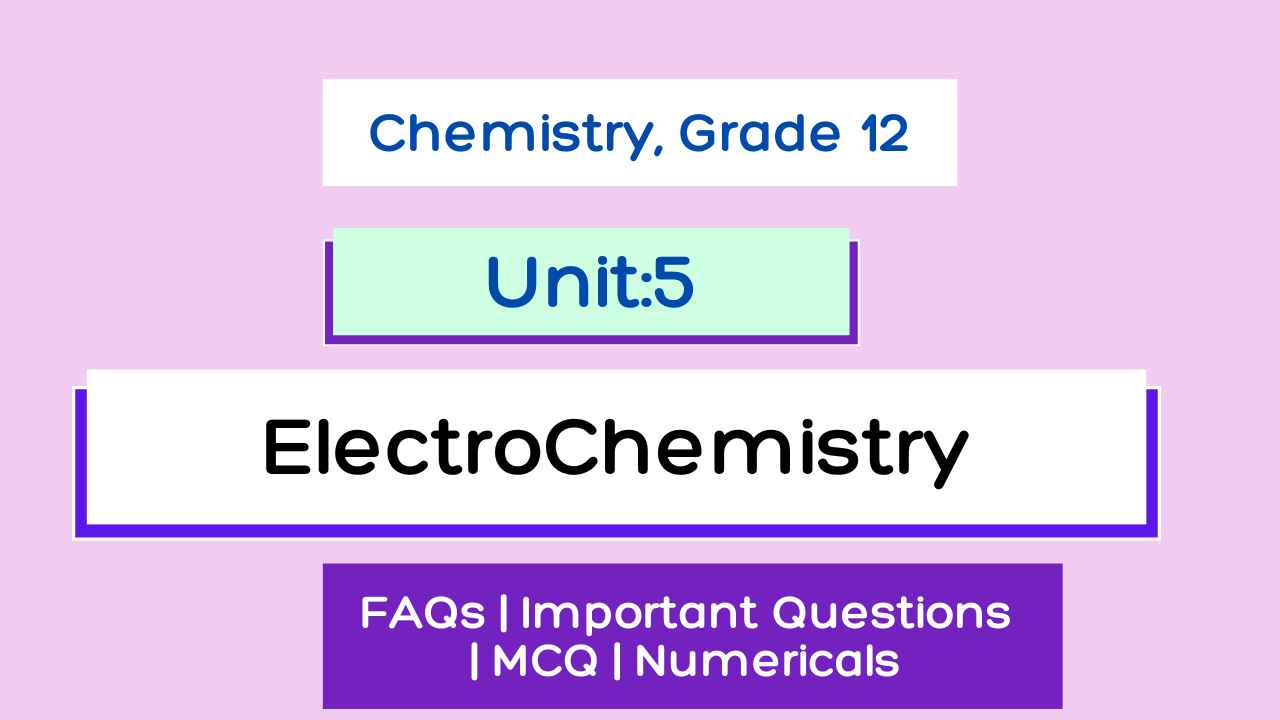Unit 5: Electrochemistry Class 12 notes

## Unit 5: Electrochemistry

Electrochemistry is the branch of chemistry that deals with the relationship between electrical energy and chemical energy and the interconversion of one form into another.

• Conversion of electrical energy into chemical change involves electrolytic reaction ( i.e. electrolysis ). Redox reaction occurs non-spontaneously.
• The conversion of chemical energy into electrical energy involves a Galvanic reaction. Redox reaction occurs spontaneously.

### Origin of Electrode potential (Single electrode potential)

When a metal rod (M) is dipped into a solution containing its own ions (Mn+)then the following three possibilities can occur.

#### 1. No Charge is developed:

Metal ions may collide with a metal rod and deflect back without any change.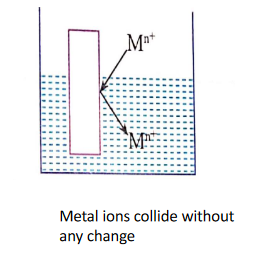#### 2. Reduction:

Metal ions, on collision with a metal rod, may gain electrons and changed into metal atoms i.e. Mn+ ions are reduced. (metal gain electron)

Mn+ +ne- → M …..(i)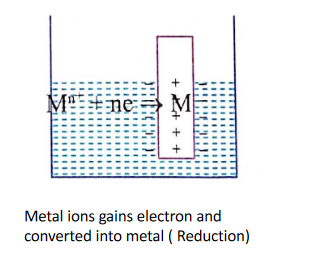#### 3. Oxidation

Metal atoms of the metal rod may lose electrons and change into Mn+ ions i.e. metal atoms get oxidized. (metal loss electron)

M → Mn+ + ne- ……..(ii)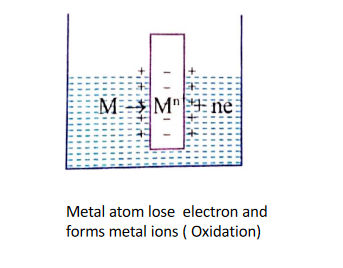#### What actually happens depends upon the relative tendency of a metal or its ions.

If the metal has a relatively greater tendency to get an oxidized reaction Eqn. (ii)(3) will occur, and the electrons will accumulate on the metal rod, which will therefore develop a negative charge with respect to the solution. This in turn may attract some metal ions from the solution which may change into metal atoms. After some time, equilibrium will establish as: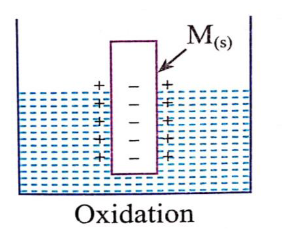Similarly, if metal ions have a greater tendency to get reduced, reaction Eqn. (i)(2) will occur. Metal ions will gain electrons from a metal rod. As a result metal rod will develop a positive charge with respect to the solution and ultimately the equilibrium will establish as: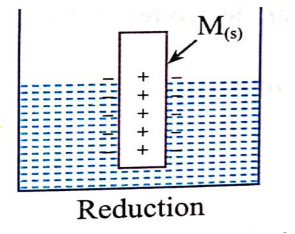Thus, in either case, there is the separation of opposite charges between the metal rod and its ions in solution which leads to the development of an electrical double layer on the metal electrode and electrolyte solution interface.

As a result, a potential difference was established between the metal and its ions in the solution interface. The potential difference thus developed is called single electrode potential.

Thus, the electrode potential is defined as the potential difference set up between a metal rod and its ions in solution due to the separation of charges between them.

Simply, it is the tendency of the electrode to lose or gain electrons when it is in contact with the solution of its ion.

If the potential developed is because of the oxidation reaction in the metal plate is called oxidation potential and if the potential developed is because of the reduction reaction in the metal plate is called reduction potential.

#### Electrode potential depends on:

1. Nature of metal electrode.
2. The concentration of metal ions in the solution.
3. The temperature of the solution.

### Standard electrode potential

If the electrode potential is measured in standard conditions i.e. 1 molar concentration (1M) of the electrolytic solution, the temperature at 250C, and 1 atm pressure (in case of gas electrode) then it is called standard electrode potential. It is denoted by E0.

It is of two types:

1. Standard oxidation electrode potential
2. Standard reduction electrode potential

#### 1. Standard oxidation electrode potential:

If Electrode potential is developed due to an oxidation reaction, it is called oxidation potential. Oxidation potential at standard conditions is called standard oxidation potential.

𝑍𝑛 (s)        𝑍𝑛2+ (𝑎𝑞) + 2𝑒−     (𝑜𝑥𝑖𝑑𝑎𝑡𝑖𝑜𝑛)         𝐸' 𝑍𝑛/𝑍𝑛2+ 0 = +0.76 𝑉
𝐶𝑢 (s)     →     𝐶𝑢2+ (𝑎𝑞) + 2𝑒−     (𝑜𝑥𝑖𝑑𝑎𝑡𝑖𝑜𝑛)         𝐸' 𝐶𝑢/𝐶𝑢2+ 0 = −0.34 𝑉
𝑀𝑔 (s)        𝑀𝑔2+ (𝑎𝑞) + 2𝑒−     (𝑜𝑥𝑖𝑑𝑎𝑡𝑖𝑜𝑛)       𝐸' 𝑀𝑔/𝑀𝑔2+ 0 = +2.37 𝑉

#### 2. Standard reduction electrode potential:

If electrode potential is developed due to a reduction reaction, it is called reduction potential. Reduction potential at standard conditions is called standard reduction potential.

𝑍𝑛2+ (𝑎𝑞) + 2𝑒−     → 𝑍𝑛  (s) (𝑟𝑒𝑑𝑢𝑐𝑡𝑖𝑜𝑛)         𝐸𝑍𝑛2+/𝑍𝑛 0 = −0.76 𝑉
𝐶𝑢2+ (𝑎𝑞) + 2𝑒−     → 𝐶𝑢  (s) (𝑟𝑒𝑑𝑢𝑐𝑡𝑖𝑜𝑛)         𝐸𝐶𝑢2+/𝐶𝑢 0 = +0.34 𝑉
𝑀𝑔2+ (𝑎𝑞) + 2𝑒−     → 𝑀𝑔 (s) (𝑟𝑒𝑑𝑢𝑐𝑡𝑖𝑜𝑛)         𝐸𝑀𝑔2+/𝑀𝑔 0 = −2.37 𝑉
Note :

1. Oxidation potential = Anode
2. Reduction potential = Cathode
3. In the IUPAC system, standard electrode potential means standard reduction electrode potential.
4. Standard reduction electrode potential value = - Standard oxidation electrode potential value.

𝐸' 𝑍𝑛2+/𝑍𝑛(reduction)= −𝐸' 𝑍𝑛/𝑍𝑛2+ (oxidation)

## Standard hydrogen electrode and Calomel electrode

### Reference Electrode:

The Electrode whose Electrode potential is known as Zero(0)  is called Reference Electrode.

### Types of Reference Electrodes

The reference electrodes are classified into two types:

1. Primary Reference Electrode: The Primary Reference Electrode is Standard Hydrogen Electrode (SHE) which Electrode Potential is Known. Example: Standard Hydrogen Electrode (SHE)
2. Secondary Reference Electrode: The electrode whose potential is determined by connecting to the standard hydrogen electrode is called a secondary reference electrode. Example: Mercury Electrode (Calomel Electrode), Ag-Ag, Gas Electrode

#### Standard Hydrogen Electrode (SHE)

The primary reference electrode for calculating the electrode potential of a cell is a standard hydrogen electrode. The absolute value of the electrode potential of a single electrode cannot be determined because oxidation half-reaction or reduction half-reaction cannot take place alone.

It consists of Platinum and HCl Solutions. Inert Nature of Pt (platinum) is used.
(Platinum is chemically inert and will not oxidize in air at any temperature)

It is measured using reference electrodes like Standard hydrogen electrodes, Calomel electrodes, etc.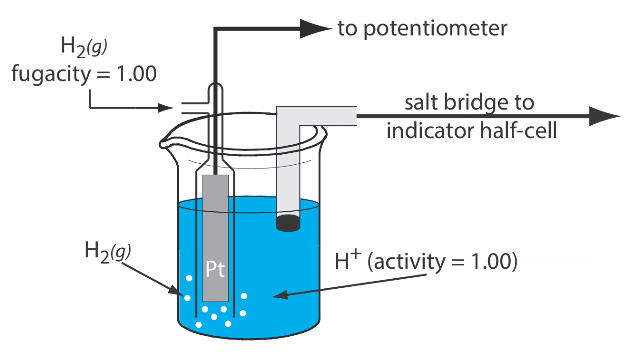Standard hydrogen electrode.

#### Calomel Electrode (Mercury Electrode)

The Secondary reference Electrode which is Standardized by Standard Hydrogen Electrode (SHE) and used as a secondary reference electrode to determine the standard potentials of the electrode is called the Calomel Electrode.

KCl, Hg, and Hg2Cl2 ( calomel) are used in this Electrode and They can behave like Oxidation and Reduction which depend upon the saturated solution of KCl (Cl-).

The electrode consists of a glass tube. A little Hg is placed at the bottom of the dry glass tube. A thin glass tube with a platinum wire is then inserted, taking care that the platinum wire drips into mercury.

The mercury is then covered with a layer of mercury and mercurous chloride (calomel) paste. The glass tube is then filled with a KCl solution of definite concentration as shown in fig.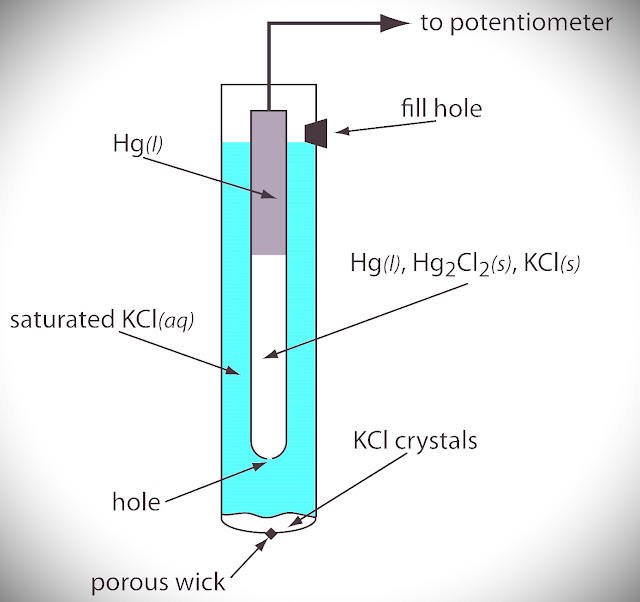Fig: Calomel Electrode

If Saturated KCl is given there, then the Calomel Electrode becomes a Saturated Calomel Electrode( SCE ).

Calculating a Standard Cell Potential

( some calculations and concepts)

### Application of Electrochemical Cell

Electrochemical series is an Arrangement of metallic elements or ions in the increasing oxidizing potential or Standard Reduction Potential.

The Applications are given as:

1. To Find the Anode and Cathode: In the electrochemical series, the positive value (+ve) is the oxidizing agent so it is the anode and the negative value (-ve) is the reducing agent so it is the cathode.
2. To Find the standard cell potential: standard cell potential is the potential difference between the cathode and anode.
3. To Find oxidizing and reducing agents: In the electrochemical series, positive values ​​(+ve) are oxidizing agents and negative values ​​(-ve) are reducing agents.
4. To Find Reactivity: Strong oxidizing agents always react with strong reducing agents, so Cu (strong oxidizing agent) reacts with Zn (strong reducing agent).
5. For Electropositive: The electrochemical series tells us how electropositive or electronegative the element/ion combination is compared to a standard hydrogen electrode.
6. Oxidizing potential for non-metals: The nature of oxidation depends on the electron or tendency to accept electrons. The higher the value of the reduction potential, the higher the tendency to accept electrons. Therefore, the oxidizing properties increase from top to bottom of the electrochemical series.
7. Reducing the potential for metals: The more negative the reduction potential, the greater the tendency to lose electrons. Therefore, reducibility decreases from top to bottom of the electrochemical series. The performance of the reducing agent increases as the standard reduction potential of the electrochemical series becomes more negative.
8. Thermal Stability: Since the Electropositive properties of metals decrease from top to bottom, the thermal stability also decreases from top to bottom.
9. Extraction of metals: Depending on the position of the metals in the electrochemical series, we can determine how to extract them from the source. Metals such as Li, K, Na, Ca, and Mg (metals on Fe) can be separated by electrolysis of molten solutions of metal chlorides.

### Electrochemical cell

An electrochemical cell is a device that can either produce electrical energy from a chemical reaction or promote a chemical reaction through the introduction of electrical energy.

### Electrolytic cell

An electrolytic cell is a chemical cell that undergoes redox reactions when an electric current is passed through it.

It is mainly used to break down compounds and this process is called hydrolysis.
They are the basis of batteries.

In the electrolyte, two electrodes are connected to an external DC circuit within the electrolytic solution.

#### Electrolytic cells are used for all kinds of things:

• Electroplating metals
• Recharging a battery
• Separating pure metals from metallic compounds etc

### Galvonic cell ( voltaic cell )

A galvanic cell (Voltaice cell) is an electrochemical cell that derives electrical energy from spontaneous redox reactions that occur in half cells connected by salt bridges or in single cells separated by a porous medium.

#### Galvanic cells ( voltaic cells)  are used for all kinds of things:

• The basis for a battery

### Difference Between Galvanic Cells And Electrolytic Cells

Electrolytic cell

Galvanic cell

i. It converts electrical energy into chemical energy.

i. It converts chemical energy into electrical energy.

ii. Redox reaction occurs non spontaneously

ii. Redox reaction occurs spontaneously.

iii. Anode is represented by +ve sign and cathode is represented by - ve sign.

iii. Anode is represented by the – ve sign and the cathode is represented by the +ve sign.

iv. Salt bridge is not used.

iv. Salt bridge is used.

v. Two electrodes are dipped in the same electrolytic solution within the same container.

v. Two electrodes are dipped in two different electrolytic solutions containing their own ion in two different containers

### Daniel Cell (galvonic) (Zn-Cu)

The Zn rods are immersed in a Zn2+ (ZnSO4) solution, while the Cu rods are immersed in a Cu2+ (CuSO4) solution. As shown in the pictures, they are connected to a U-tube containing KCl, NH4Cl in gelatin, or a semi-solid paste of agar-salt bridges. This cell is known as a Daniel (galvanic) (Zn-Cu) cell.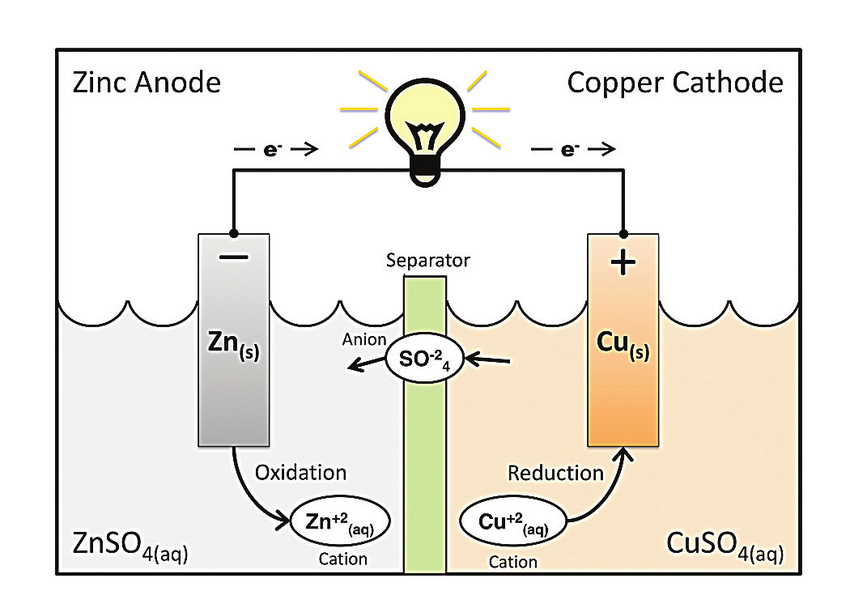Daniel (galvanic) (Zn-Cu) cell

(i) At the zinc electrode, the metal undergoes oxidation and gives 2 electrons ( de-electronation).

Zn(s) Zn++ ( aq) + 2e-

so, Zinc acts as a negative, anode.

(ii) At the copper electrode, the metal undergoes reduction which needs electrons( electronation)

Cu++(aq) + 2e-  Cu(s)

so, copper acts as a positive, cathode.

Overall reaction:
Zn(s) + cu++(aq)   Zn++(aq) + Cu (s)
At anode: Zn(s)   Zn++ ( aq) + 2e-  ( oxidation )
At cathode: Cu++(aq) + 2e-  Cu(s) ( reduction )
Note: Because electrons are discharged at the anode, it gains negative polarity, whereas the cathode gains positive polarity because it requires electrons.
Cell Notation:
Zn / Zn ++ // Cu ++ / Cu

### Direction of Electron

• In a galvanic cell: The cathode is positively charged, whereas the anode is negatively charged. Electrons enter the solution from the positive cathode.
• In an electrolytic cell: Anode is positive while the cathode is negative. The cathode becomes negative when an electron from an external source enters it, and the electrode becomes positive when an electron from the anode enters the external power source.
( Oxidation = e- deduction, Reduction = e- addition )

## Predicting the Feasibility of a redox reaction

### With the help of the electrochemical series,

The net emf of the cell reaction Ecell can be calculated from the expression:
E° cell =E° cathode - E°anode
E' cell = +ve, the reaction is feasible.
E' cell = -ve, the reaction is not feasible.

Haloalkanes Class 12 Chemistry notes NEB

### NERNST Equation For Electrode And Cell Potential

(:) The standard cell potential, temperature, and reaction quotient are all related by the NERNST Equation to the cell potential of an electrochemical cell. The Nernst equation can be used to calculate electrochemical cells' cell potentials even under exceptional situations.

It is used to calculate the cell potential of an electrochemical cell at any given temperature, pressure, and reactant concentration.

### NERNST EQN:

E cell = E° cell - (RT/nF)lnQ

E° cell = Cell potential under standard Concentration.
E cell = Cell potential of the cell.
R = universal gas constant ( 8.31 JK-1 mol-1 )
T= Temperature ( 298 °K ) ( 25℃ )
n= number of electrons transferred in the redox reaction
F= faraday's Constant ( (96500 Columb mol -1)
Q= Reactant quotient

The relationship between standard free energy change and standard cell potential

ΔG°cell = −nFE°
ΔG°cell=Gibbs free energy

In a galvanic cell, the Gibbs free energy is related to the potential by ΔG°cell = −nFE° cell.

If E°cell > 0, then the process is spontaneous (galvanic cell).

If E°cell < 0, then the process is non-spontaneous (electrolytic cell).

### Commercial Cell

• Light in weight and compact size
• produce constant voltage during use

Commercial cells are also electrochemical cells, however not all electrochemical cells are commercial cells since not every redox reaction is suited for developing commercial cells with salt bridges, high internal resistance, and transportation.

Commercial cells should be portable and have minimal voltage loss while in use.

A battery is a collection of two or more cells connected in series.

#### A commercial cell is defined as follows:

1. Primary Cell
2. Secondary cell
3. Fuel cell

### 1. Primary cell

An external electric energy source cannot reverse the electrode reactions in these cells. Reactions occur just once in these cells, and they expire after usage. As a result, they are not chargeable.
Dry cells & button cells are two common examples.

#### Dry Cells

A dry cell is a type of electric battery, commonly used for portable electrical devices.

##### Inventor: French ER.Georges Leclanché

These are found in flashlights, toys, flashlights, calculators, tape recorders, and a variety of other gadgets.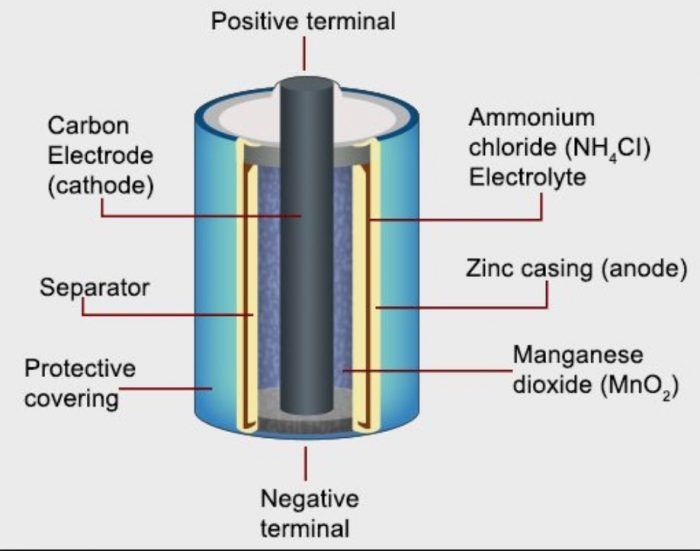An image of a Dry Cell

#### Dry Cell Construction

a) It is made up of a zinc cylinder. This is filled with a moist NH4Cl paste and a little amount of ZnCl2.

b) A zinc cylinder serves as an anode. A graphite (carbon) rod serves as the cathode.

c) A black paste of manganese dioxide (MnO2) and carbon powder surrounds the carbon rod.

d) During usage, the zinc casing is consumed, and as a result, it develops holes that cause leaks. An iron or steel sheet covers the zinc in leak-resistant or dry cells.

Zn/Zn ++ // NH4 / NH3 +

The reactions occurring at the electrodes are as follows:

Anode

Zn —⟶ Zn2+ + 2e‾

Cathode

NH4+(aq) + MnO2(s) +2e¯ ——⟶ MnO(OH) + NH3

—————————————————————-

Zn + 2NH4+ (aq) + 2MnO2(s) ——⟶Zn2+ + 2MnO(OH) +2NH3

### 2. Secondary cells

Some cells can be recharged. These are referred to as batteries or storage cells. These can be recharged and reused as a source of electric power again and again.

This cell is also rechargeable. It lasts longer than lead storage cells but is more costly than lead storage batteries. However, because it is smaller and lighter, it offers some advantages. It is suitable for use in portable and cordless products.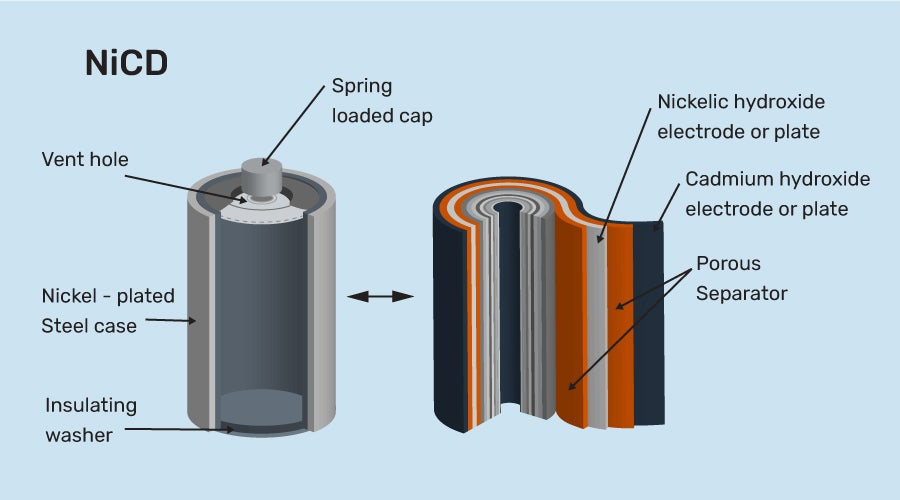Nickel Cadmium Storage Cell

##### Nickel Cadmium Storage Cell Construction

a) It is made up of a cadmium anode and a metal grid containing NiO2 that serves as a cathode.

b) KOH is the electrolyte in this cell.

The reactions occurring at the electrodes are as follows:

Anode

Cd(s)+2OH¯ ⇔ CdO(s) +H2O(l)+ 2e¯

Cathode

2Ni(OH3) (s) + 2e¯ ⇔ 2Ni(OH)2(s) +2OH¯ (aq)

——————————————————–

Cd(s)+ 2Ni(OH3) (s) ⇔ CdO(s) + 2Ni(OH)2(s) + H2O(l)

### 3. Fuel Cell

A fuel cell is an electrical energy-producing device that transforms chemical energy. Fuel cells, like batteries, require a constant supply of fuel, most often hydrogen. They will continue to generate energy as long as there is fuel available.

Satellites, space capsules, autos, boats, and submarines have all been powered by hydrogen fuel cells.

Examples of fuel cells include biochemical oxygen fuel cells, hydrogen-oxygen fuel cells, propane-oxygen fuel cells, methyl alcohol fuel cells, and alkaline fuel cells.In this hydrogen fuel-cell schematic, oxygen from the air reacts with hydrogen, producing water and electricity. source: wikimedia.org

#### Fuel cell Construction

1) In this cell, hydrogen and oxygen are bubbled into concentrated aqueous sodium hydroxide or potassium hydroxide via a porous carbon electrode.

2) The fuel, hydrogen, is supplied into the anode compartment and oxidized.

3) The oxygen is supplied into the cathode compartment and reduced.

The reactions occurring at the electrodes are as follows:

Anode

2H2+2O2−⟶2H2O+4e−

Cathode

O2+4e−⟶2O2−

——————————————————–

2H2+O2⟶2H2O

Batteries are collections of galvanic cells or cells that generate an electric current. The potential of a battery formed from numerous cells is an integer multiple of the potential of each individual cell.

Batteries may be divided into two categories: primary and secondary. Since they are "single use," primary batteries cannot be recharged. Examples of primary batteries include dry cells and (most) alkaline batteries.

A secondary battery is the second kind, which is rechargeable. Lead acid, lithium-ion, and nickel-cadmium (NiCd) batteries are a few types of secondary batteries.

In that they produce an electrical current, fuel cells and batteries are similar in that both require constant additions of fuel and oxidizer.

Tags

• Electrochemistry class 12 notes neb
• asmita old is gold solution grade 12 chemistry
• class 12 chemistry notes nepal
• class 12 chemistry solutions neb pdf
• class 12 organic chemistry notes pdf

Getting Info...